# Assessing the Proportional Odds Assumption and Its Impact

2022
accuracy-score
dichotomization
endpoints
ordinal
This article demonstrates how the proportional odds (PO) assumption and its impact can be assessed. General robustness to non-PO on either a main variable of interest or on an adjustment covariate are exemplified. Advantages of a continuous Bayesian blend of PO and non-PO are also discussed.
Author
Affiliation

Department of Biostatistics
Vanderbilt University School of Medicine

Published

March 9, 2022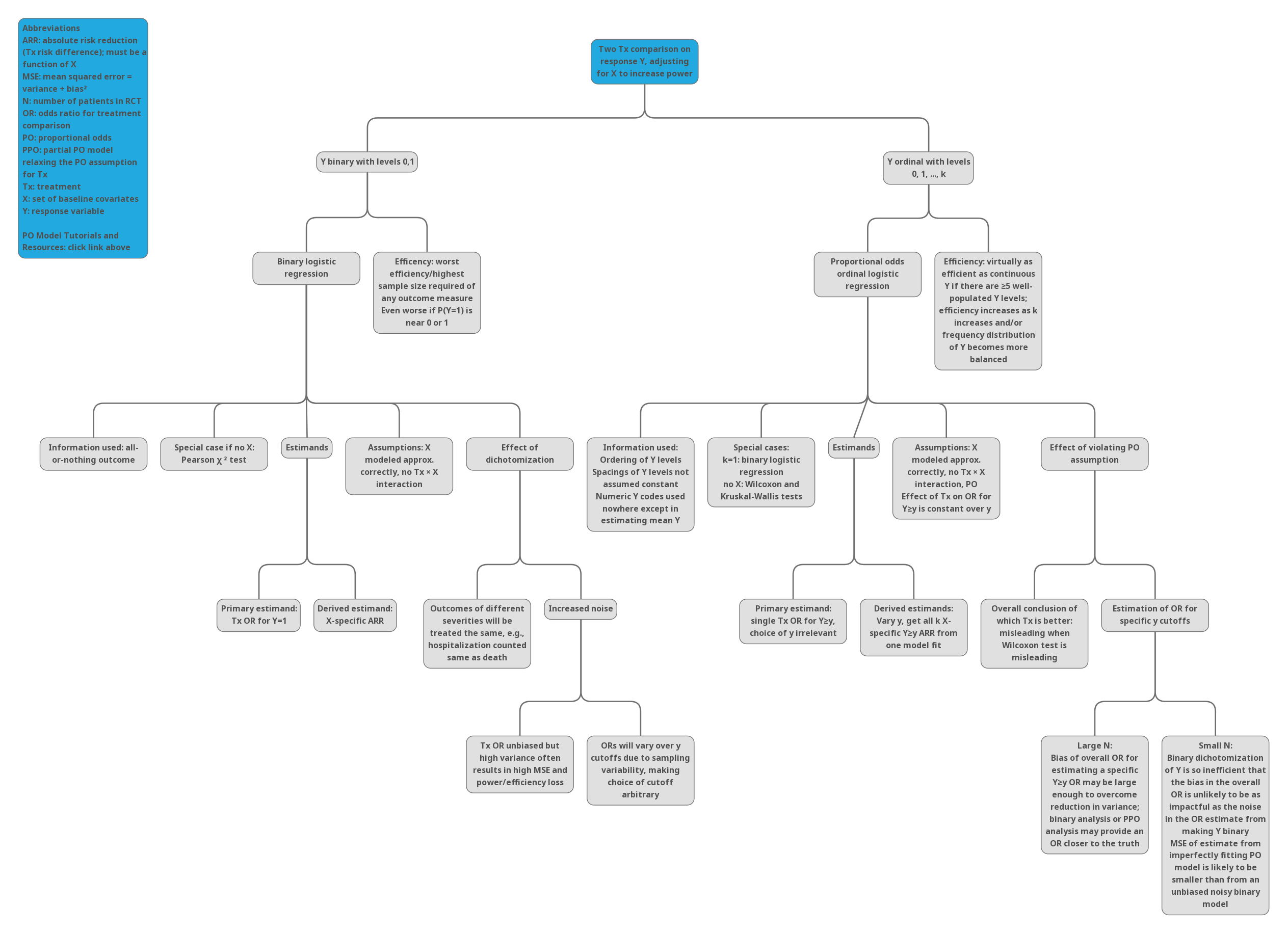# Introduction

Reviewers who do not seem to worry about the proportional hazards assumption in a Cox model or the equal variance assumption in a $$t$$-test seem to worry a good deal about the proportional odds (PO) assumption in a semiparametric ordinal logistic regression model. This in spite of the fact that proportional hazards and equal variance in other models are exact analogies to the PO assumption. Furthermore, when there is no covariate adjustment, the PO model is equivalent to the Wilcoxon test, and reviewers do not typically criticize the Wilcoxon test or realize that it has optimum power only under the PO assumption.

The purpose of this report is to (1) demonstrate examinations of the PO assumption for a treatment effect in a two-treatment observational comparison, and (2) discuss various issues around PO model analysis and alternative analyses using cutpoints on the outcome variable. It is shown that exercises such as comparing predicted vs. observed values can be misleading when the sample size is not very large.

# Dataset

The dataset, taken from a real observational study, consists of a 7-level ordinal outcome variable y having values 0-6, a treatment variable trt, and a strong baseline variable baseline defined by a disease scale that is related to y but with more resolution. This is a dominating covariate, and failure to adjust for it will result in a weaker treatment comparison. trt levels are A and B, with 48 patients given treatment B and 100 given treatment A.

Code
getHdata(txpo)
d <- txpo
if(ishtml) html(describe(d)) else latex(describe(d), file='')
d Descriptives
d

3 Variables   148 Observations

y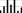nmissingdistinctInfoMeanGmd
148070.95431.759
lowest : 0 1 2 3 4 , highest: 2 3 4 5 6
 Value          0     1     2     3     4     5     6
Frequency      6    19    39    24    40     5    15
Proportion 0.041 0.128 0.264 0.162 0.270 0.034 0.101


trt
nmissingdistinct
14802
 Value          A     B
Frequency    100    48
Proportion 0.676 0.324


baseline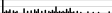nmissingdistinctInfoMeanGmd.05.10.25.50.75.90.95
1480370.99320.3117.63 0 0 420323842
lowest : 0 1 2 3 4 , highest: 44 48 51 54 60
Code
with(d, pr(obj=table(trt, y)))
   y
trt  0  1  2  3  4  5  6
A  4  8 26 19 27  2 14
B  2 11 13  5 13  3  1

# Proportional Odds Model

Code
f <- lrm(y ~ trt + baseline, data=d)
f

Logistic Regression Model

 lrm(formula = y ~ trt + baseline, data = d)


Frequencies of Responses

  0  1  2  3  4  5  6
6 19 39 24 40  5 15

Model Likelihood
Ratio Test
Discrimination
Indexes
Rank Discrim.
Indexes
Obs 148 LR χ2 69.26 R2 0.386 C 0.778
max |∂log L/∂β| 4×10-13 d.f. 2 R22,148 0.365 Dxy 0.556
Pr(>χ2) <0.0001 R22,141.3 0.379 γ 0.571
Brier 0.151 τa 0.449
β S.E. Wald Z Pr(>|Z|)
y≥1   6.1565  0.6167 9.98 <0.0001
y≥2   4.3821  0.4718 9.29 <0.0001
y≥3   2.5139  0.3600 6.98 <0.0001
y≥4   1.5520  0.3174 4.89 <0.0001
y≥5  -0.3033  0.3150 -0.96 0.3357
y≥6  -0.6738  0.3361 -2.00 0.0450
trt=B  -1.1328  0.3290 -3.44 0.0006
baseline  -0.0888  0.0121 -7.32 <0.0001
Code
summary(f)
 Low High Δ Effect S.E. Lower 0.95 Upper 0.95 Effects   Response: y baseline 4 32 28 -2.48800 0.3397 -3.15300 -1.8220 Odds Ratio 4 32 28 0.08311 0.04271 0.1617 trt — B:A 1 2 -1.13300 0.3290 -1.77800 -0.4880 Odds Ratio 1 2 0.32210 0.16900 0.6138
Code
anova(f)
 χ2 d.f. P Wald Statistics for y trt 11.86 1 0.0006 baseline 53.63 1 <0.0001 TOTAL 56.57 2 <0.0001

# Volatility of ORs Using Different Cutoffs

Even when the data generating mechanism is exactly proportional odds for treatment, different cutoffs of the response variable Y can lead to much different ORs when the sample size is not in the thousands. This is just the play of chance (sampling variation). To illustrate this point, consider the observed proportions of Y for trt=A as population probabilities for A. Apply an odds ratio of 0.3 to get the population distribution of Y for treated patients. For 10 simulated trials, sample from these two multinomial distributions and compute sample ORs for all Y cutoffs.

Code
p <- table(d$y[d$trt == 'A'])
p <- p / sum(p)
p   # probabilities for SOC

0    1    2    3    4    5    6
0.04 0.08 0.26 0.19 0.27 0.02 0.14 
Code
set.seed(1)
round(simPOcuts(n=210, odds.ratio=0.3, p=p), 2)
              y>=1 y>=2 y>=3 y>=4 y>=5 y>=6
Simulation 1  0.13 0.25 0.33 0.28 0.14 0.16
Simulation 2  0.39 0.26 0.41 0.41 1.00 1.00
Simulation 3  0.39 0.37 0.44 0.47 0.90 0.78
Simulation 4  0.18 0.27 0.30 0.41 0.32 0.23
Simulation 5  0.43 0.31 0.24 0.17 0.43 0.33
Simulation 6  0.17 0.37 0.37 0.39 0.36 0.30
Simulation 7  0.14 0.24 0.22 0.17 0.30 0.33
Simulation 8  0.37 0.50 0.28 0.29 0.26 0.26
Simulation 9  0.85 0.45 0.36 0.40 0.54 0.61
Simulation 10 0.36 0.34 0.31 0.26 0.21 0.22

See here for simPOcuts source code.

# Examining the PO Assumption

For discrete Y we are interested in checking the impact of the PO assumption on predicted probabilities for all of the Y categories, while also allowing for covariate adjustment. This can be done using the following steps:

• Select a set of covariate settings over which to evaluate accuracy of predictions
• Vary at least one of the predictors, i.e., the one for which you want to assess the impact of the PO assumption
• Fit a PO model the usual way
• Fit models that relaxes the PO assumption
• to relax the PO assumption for all predictors fit a multinomial logistic model
• to relax the PO assumption for a subset of predictors fit a partial PO (PPO) model
• For all the covariate combinations evaluate predicted probabilities for all levels of Y using the PO model and the relaxed assumption models
• Use the bootstrap to compute confidence intervals for the differences in predicted values between a PO model and a relaxed model. This will put the differences in the right context by accounting for uncertainties. This guards against over-emphasis of differences when the sample size does not support estimation, especially for the relaxed model with more parameters. Note that the same problem occurs when comparing predicted unadjusted probabilities to observed proportions, as observed proportions can be noisy.

Level 5 of y has only 5 patients so we combine it with level 6 for fitting the two relaxed models that depend on individual cell frequencies. Similarly, level 0 has only 6 patients, so we combine it with level 1. The PPO model is fitted with the VGAM R package, and the nonpo argument below signifies that the PO assumption is only being relaxed for the treatment effect. The multinomial model allows not only non-PO for trt but also for baseline. See here for impactPO source code.

Code
nd <- data.frame(trt=levels(d$trt), baseline=4) d$y5 <- with(d, pmin(pmax(y, 1), 5))
w <- impactPO(y5 ~ trt + baseline, nonpo = ~ trt,
data=d, newdata=nd, B=300)
w
                          PO     PPO    Multinomial
Deviance                  395.58 393.10 388.36
d.f.                       6      9     12
AIC                       407.58 411.10 412.36
p                         2      5      8
LR chi^2                  69.41  71.89  76.63
LR - p                    67.41  66.89  68.63
LR chi^2 test for PO             2.48   7.22
d.f.                            3      6
Pr(>chi^2)                     0.4792 0.3013
MCS R2                    0.374  0.385  0.404
MCS R2 adj                0.366  0.364  0.371
McFadden R2               0.149  0.155  0.165
Mean |difference| from PO        0.021  0.042

Covariate combination-specific mean |difference| in predicted probabilities

method trt baseline Mean |difference|
1          PPO   A        4             0.010
2          PPO   B        4             0.033
11 Multinomial   A        4             0.032
21 Multinomial   B        4             0.052

Bootstrap 0.95 confidence intervals for differences in model predicted
probabilities based on 300 bootstraps

trt baseline
1   A        4

PO - PPO probability estimates

1      2      3      4      5
Lower -0.004 -0.017 -0.058 -0.055 -0.042
Upper  0.008  0.019  0.008  0.081  0.058

PO - Multinomial probability estimates

1      2      3      4      5
Lower 0.002 -0.017 -0.152 -0.105 -0.037
Upper 0.020  0.071 -0.006  0.107  0.133

trt baseline
2   B        4

PO - PPO probability estimates

1      2      3      4      5
Lower -0.043 -0.077 -0.025 -0.191 -0.102
Upper  0.013  0.083  0.197  0.065  0.095

PO - Multinomial probability estimates

1      2      3      4      5
Lower -0.050 -0.025 -0.051 -0.272 -0.143
Upper  0.035  0.147  0.194  0.041  0.095

Comparisons of the PO model fit with models that relax the PO assumption above can be summarized as follows.

• By AIC, the model that is most likely to have the best cross-validation performance is the fully PO model (the lower the AIC the better)
• There is no evidence for non-PO, either when judging against a model that relaxes the PO assumption for treatment (P=0.48) or against a multinomial logistic model that does not assume PO for any variables (P=0.30).
• The McFadden adjusted $$R^2$$ index, in line with AIC, indicates the best fit is from the PO model
• The Maddala-Cox-Snell adjusted $$R^2$$ indicates the PO model is competitive. See this for information about general adjusted $$R^2$$ measures.
• Nonparametric bootstrap percentile confidence intervals for the difference in predicted values between the PO model and one of the relaxed models take into account uncertainties and correlations of both sets of estimates. In all cases the confidence intervals are quite wide and include 0 (except for one case, where the lower confidence limit is 0.002), which is very much in line with apparent differences being clouded by overfitting (high number of parameters in non-PO models).

These assessments must be kept in mind when interpreting the inter-model agreement between probabilities of all levels of the ordinal outcome in the graphic that follows. According to AIC and adjusted $$R^2$$, the estimates from the partial PO model and especially those from the multinomial model are overfitted. This is related to the issue that odds ratios computed from oversimplifying an ordinal response by dichotomizing it are noisy (also see the next to last section below).

Code
revo <- function(z) {
z <- as.factor(z)
factor(z, levels=rev(levels(as.factor(z))))
}
concord <- W / prod(table(d$trt)) Code u <- lrm(y ~ trt, data=d) u Logistic Regression Model  lrm(formula = y ~ trt, data = d)  Frequencies of Responses  0 1 2 3 4 5 6 6 19 39 24 40 5 15  Model Likelihood Ratio Test Discrimination Indexes Rank Discrim. Indexes Obs 148 LR χ2 4.18 R2 0.029 C 0.555 max |∂log L/∂β| 2×10-7 d.f. 1 R21,148 0.021 Dxy 0.110 Pr(>χ2) 0.0409 R21,141.3 0.022 γ 0.247 Brier 0.240 τa 0.088 β S.E. Wald Z Pr(>|Z|) y≥1 3.4217 0.4390 7.79 <0.0001 y≥2 1.8302 0.2524 7.25 <0.0001 y≥3 0.4742 0.1948 2.43 0.0149 y≥4 -0.1890 0.1929 -0.98 0.3272 y≥5 -1.6691 0.2561 -6.52 <0.0001 y≥6 -1.9983 0.2858 -6.99 <0.0001 trt=B -0.6456 0.3174 -2.03 0.0420 Note that the $$C$$ statistic in the above table handles ties differently than the concordance probability we are interested in here. Code or <- exp(- coef(u)['trt=B']) cat('Concordance probability from Wilcoxon statistic: ', concord, '\n', 'Concordance probability estimated from OR: ', or ^ 0.65 / (1 + or ^ 0.65), '\n', sep='') Concordance probability from Wilcoxon statistic: 0.6002083 Concordance probability estimated from OR: 0.6033931 In the absence of adjustment covariates, the treatment odds ratio estimate from a PO model is essentially the Wilcoxon statistic whether or not PO holds. Many statisticians are comfortable with using the Wilcoxon statistic for judging which treatment is better overall, e.g., which treatment tends to move responses towards the favorable end of the scale. So one can seldom go wrong in using the PO model to judge which treatment is better, even when PO does not hold. ## Simulation Study of Effect of Adjusting for a Highly Non-PO Covariate What if the treatment operates in PO but an important covariate strongly violates its PO assumption? Let’s find out by simulating a specific departure from PO for a binary covariate. For a discrete ordinal outcome with levels 0,1,…,6 let the intercepts corresponding to $$Y=1, ..., 6$$ be $$\alpha = [4.4, 2.6, 0.7, -0.2, -2, -2.4]$$. Let the true treatment effect be $$\beta=-1.0$$. The simulated covariate $$X$$ is binary with a prevalence of $$\frac{1}{2}$$. The true effect of $$X$$ is to have an OR of 3.0 on $$Y\geq 1$$, $$Y\geq 2$$, $$Y\geq 3$$ but to have an OR of $$\frac{1}{3}$$ on $$Y\geq 4$$, $$Y\geq 5$$ and $$Y=6$$. So the initial regression coefficient for $$X$$ is $$\log(3)$$ and the additional effect of $$X$$ on $$Y\geq y$$ once $$y$$ crosses to 4 and above is a decrement in its prevailing log odds by $$2\log(3)$$. So here is our model to simulate from: $\Pr(Y \geq y | \mathrm{trt}, X) = \mathrm{expit}(\alpha_{y} - [\mathrm{trt=B}] + \log(3) X -2\log(3) X [y \geq 4])$ Over simulations compare these three estimates and their standard error: • unadjusted treatment effect • treatment effect adjusted for covariate assuming both treatment and covariate act in PO • treatment effect adjusted for covariate assuming treatment is PO but allowing the covariate to be arbitrarily non-PO To test the simulation, simulate a very large sample size of n=50,000 and examine the coefficient estimates from the correct partial PO model and from two other models. Code sim <- function(beta, n, nsim=100) { tx <- c(rep(0, n/2), rep(1, n/2)) x <- c(rep(0, n/4), rep(1, n/4), rep(0, n/4), rep(1, n/4)) # Construct a matrix of logits of cumulative probabilities L <- matrix(alpha, nrow=n, ncol=6, byrow=TRUE) L[tx == 1,] <- L[tx == 1, ] + beta L[x == 1, ] <- L[x == 1, ] + log(3) L[x == 1, 4:6] <- L[x == 1, 4:6] - 2 * log(3) P <- plogis(L) # cumulative probs P <- cbind(1, P) - cbind(P, 0) # cell probs (each row sums to 1.0) b <- v <- pv <- matrix(NA, nrow=nsim, ncol=3) colnames(b) <- colnames(v) <- colnames(pv) <- c('PPO', 'PO', 'No X') y <- integer(n) a <- 'tx' msim <- 0 for(i in 1 : nsim) { for(j in 1 : n) y[j] <- sample(0:6, 1, prob=P[j, ]) f <- try(vglm(y ~ tx + x, cumulative(reverse=TRUE, parallel=FALSE ~ x))) if(inherits(f, 'try-error')) next msim <- msim + 1 g <- lrm(y ~ tx + x) h <- lrm(y ~ tx) co <- c(coef(f)[a], coef(g)[a], coef(h)[a]) vs <- c(vcov(f)[a,a], vcov(g)[a,a], vcov(h)[a,a]) b[msim, ] <- co v[msim, ] <- vs pv[msim, ] <- 2 * pnorm(- abs(co / sqrt(vs))) } b <- b [1:msim,, drop=FALSE] v <- v [1:msim,, drop=FALSE] pv <- pv[1:msim,, drop=FALSE] bbar <- apply(b, 2, mean) bmed <- apply(b, 2, median) bse <- sqrt(apply(v, 2, mean)) bsemed <- sqrt(apply(v, 2, median)) sd <- if(msim < 2) rep(NA, 3) else sqrt(diag(cov(b))) pow <- if(nsim < 2) rep(NA, 3) else apply(pv, 2, function(x) mean(x < 0.05)) list(summary=cbind('Mean beta' = bbar, 'Median beta' = bmed, 'Sqrt mean estimated var' = bse, 'Median estimated SE' = bsemed, 'Empirical SD' = sd, 'Power' = pow), sims=list(beta=b, variance=v, p=pv), nsim=msim) } require(VGAM) alpha <- c(4.4, 2.6, 0.7, -0.2, -2, -2.4) set.seed(1) si <- sim(beta=-1, 50000, 1) round(si$summary, 4)
     Mean beta Median beta Sqrt mean estimated var Median estimated SE
PPO    -0.9832     -0.9832                  0.0094              0.0094
PO     -0.9271     -0.9271                  0.0168              0.0168
No X   -0.9280     -0.9280                  0.0168              0.0168
Empirical SD Power
PPO            NA    NA
PO             NA    NA
No X           NA    NA

With n=50,000 extreme non-PO in the binary covariate hardly affected the estimated treatment and its standard error, and did not affect the ratio of the coefficient estimate to its standard error. Non-PO in $$X$$ does effect the intercepts which has an implication in estimating absolute effects (unlike the treatment OR). But by examining the intercepts when the covariate is omitted entirely one can see that the problems with the intercepts when PO is forced are no worse than just ignoring the covariate altogether (not shown here).

Now simulate 2000 trials with n=300 and study how the various models perform.

Code
set.seed(7)
fi <- '~/data/sim/simtx.rds'
if(file.exists(fi)) simr <- readRDS(fi) else {
s  <- sim(-1, 300, 2000)
s0 <- sim( 0, 300, 2000)  # also simulate under the null
simr <- list(s=s, s0=s0)
saveRDS(simr, fi)
}
cat('Convergence in', simr$s$nsim, 'simulations\n\n')
Convergence in 1947 simulations
Code
kab(round(simr$s$summary, 4))
Mean beta Median beta Sqrt mean estimated var Median estimated SE Empirical SD Power
PPO -1.0157 -1.0100 0.2273 0.2281 0.2340 0.9979
PO -0.9609 -0.9565 0.2189 0.2184 0.2227 0.9974
No X -0.9599 -0.9556 0.2188 0.2183 0.2227 0.9974

The second line of the summary shows what to expect when fitting a PO model in the presence of severe non-PO for an important covariate. The mean estimated treatment effect is the same as not adjusting for the covariate and so is its estimated standard error. Both are close to the estimate from the proper model—the partial PO model that allows for different effects of $$X$$ over the categories of $$Y$$. And for all three models the standard error of the treatment effect estimated from that model’s information matrix is very accurate as judged by the closeness to the empirical SD of the simulated regression coefficient estimates.

Check simulations under the null, i.e., with $$\beta=0$$ for treatment. Look at the distribution of p-values for the three model’s treatment 2-sided Wald tests (which should be uniform), and the empirical $$\alpha$$, the fraction of Wald p-values $$< 0.05$$.

Code
p <- simr$s0$sims$p par(mfrow=c(2,2)) for(i in 1 : 3) { pow <- paste0('Fraction P<0.05: ', round(mean(p[, i] < 0.05), 3)) hist(p[, i], main=paste(colnames(p)[i], ' ', pow), xlab='P-value', sub=, nclass=50) }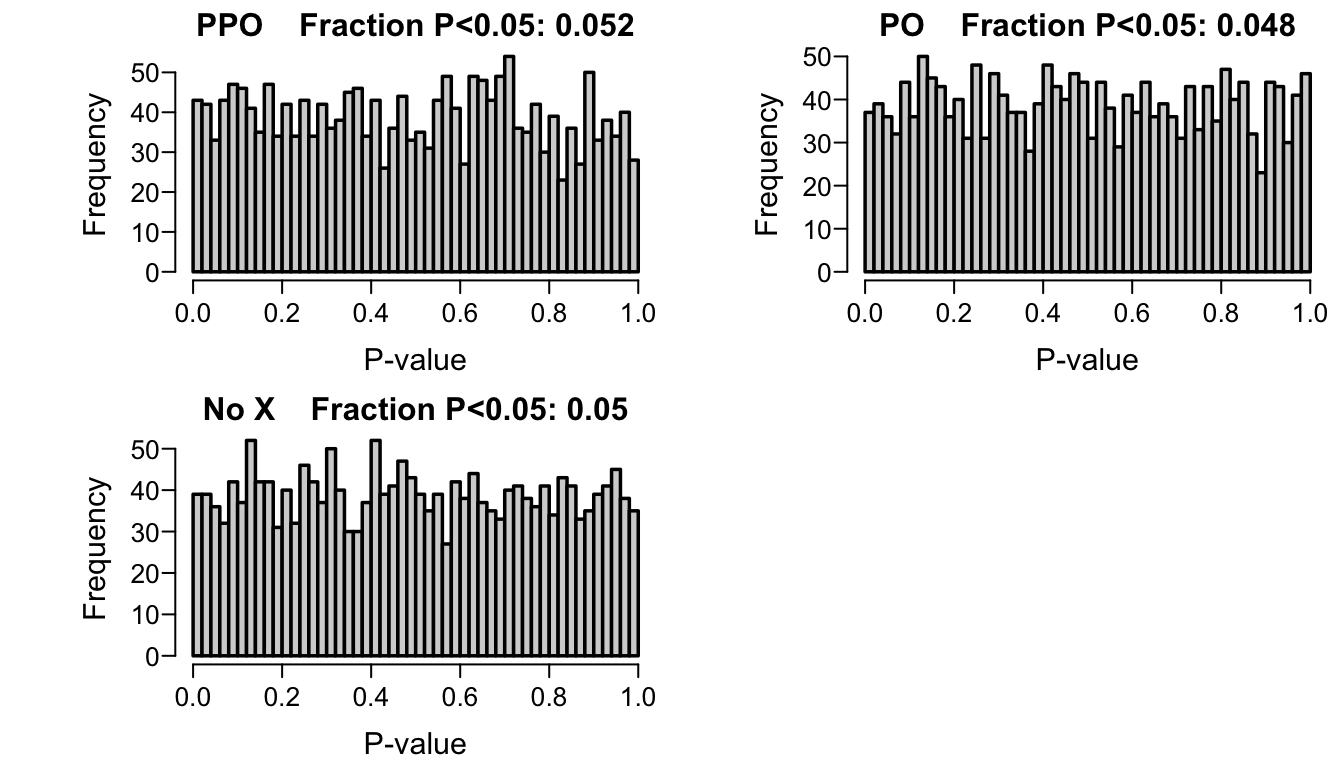This is better shown using empirical cumulative distributions. Code z <- NULL for(i in 1 : 3) { pow <- paste0('Fraction P<0.05: ', round(mean(p[, i] < 0.05), 3)) ti <- paste(colnames(p)[i], ' ', pow) z <- rbind(z, data.frame(Assumed Covariate Effect=ti, p=p[, i], check.names=FALSE)) } ggplot(z, aes(x=p, color=Assumed Covariate Effect)) + stat_ecdf(geom='step', pad=FALSE) + xlab('P-value') + ylab('Cumulative Probability')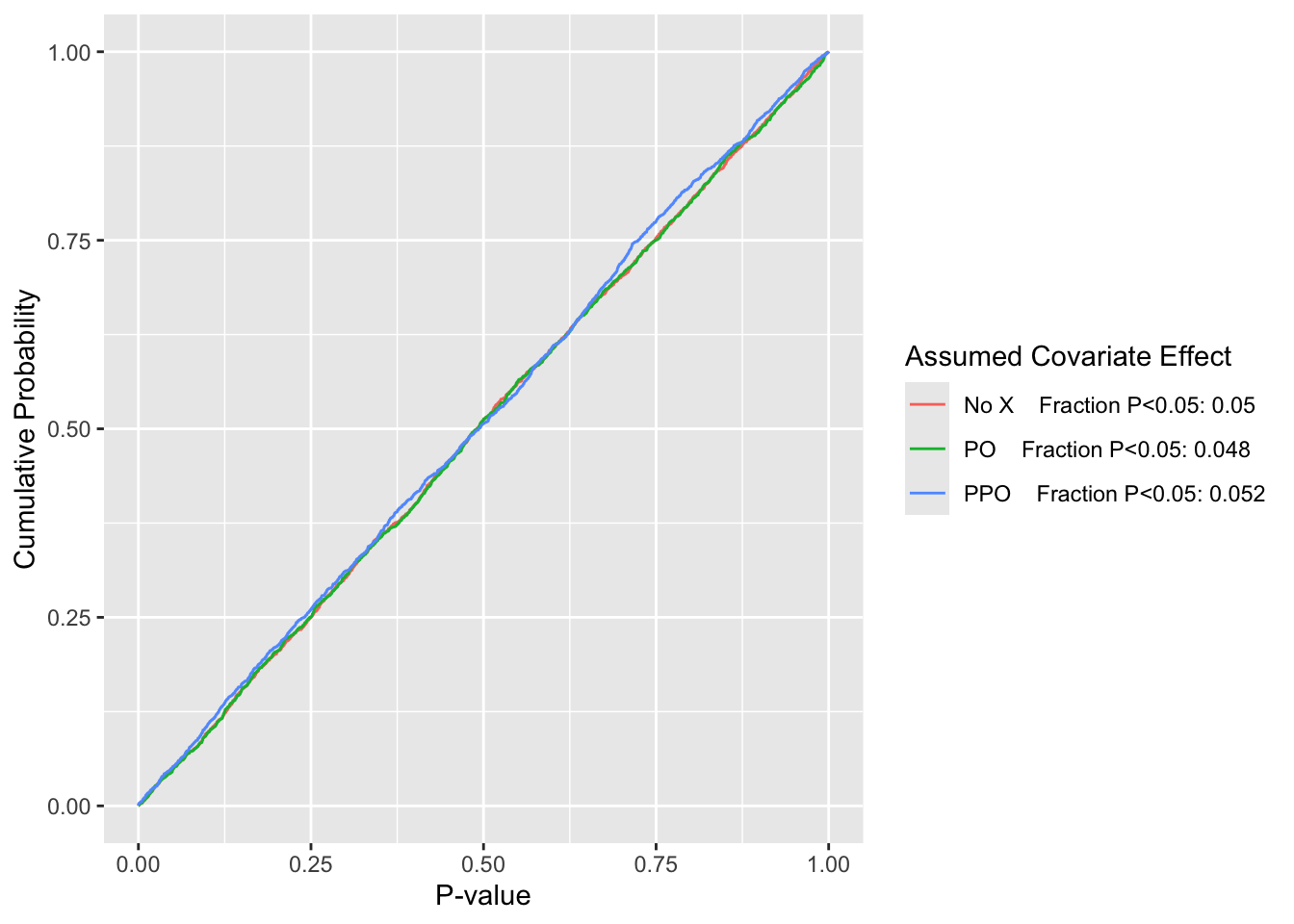Empirical cumulative distributions of p-values from Wald tests of the treatment effect under the null $$\beta=0$$, with simulation estimates of $$\alpha$$ for three models for a strong covariate $$X$$ $$\alpha$$ under the improper (with respect to $$X$$) PO model is just under that of the model ignoring $$X$$, which is estimated to be at the nominal 0.05. The estimated $$\alpha$$ for the appropriate partial PO model is just over 0.05. ## Using the PO Model to Estimate the Treatment Effect for a Specific Y Cutoff Just as in the case where one thinks that a sex by treatment interaction may be present, actually estimating such an interaction effect can make treatment estimates worse for both sexes in small samples even when the interaction is truly present. This is because estimating an unknown quantity well requires both minimal bias and good precision (low variance), and adding a parameter to the model increases variance (one must estimate both the main effect and the interaction, equivalent to estimating separate treatment effects for females and males). The probability that an estimate is within a given tolerance of the true value is closely related to the mean squared error (MSE) of the estimator. MSE equals variance plus the square of bias. Bias is the systematic error that can result from model misspecification, e.g., fitting a common OR (assuming PO) when the treatment OR needs to vary for some levels of Y (non-PO). A log odds ratio estimate for a specific cutoff Y≥y derived from a model that dichotomized the raw data at y will tend to be unbiased for estimating that specific log odds ratio. Suppose the log OR has variance $$u$$. The MSE of the log OR estimate is $$u$$ since the bias is approximately zero. Now consider estimating the common OR in a PO model and using that to estimate the OR for Y≥y. Suppose that common log OR has variance $$v$$ and bias $$b$$ ($$b$$ is a weighted log OR the PO model estimates minus the true log OR for Y≥y) so that MSE of the log OR for the PO model is $$v + b^2$$. The multiplicative bias (fold-change bias) is $$e^b$$. How large must this multiplicative bias in the OR estimate be (i.e., how much non-PO needs to exist) before the tailored model for Y≥y has lower mean squared error (on the log scale) than the less-well-fitting PO model? By comparing the two MSEs of $$u$$ and $$v + b^2$$ we find that the critical multiplicative error in the OR is $$\exp(\sqrt{u - v})$$. For the dataset we have been analyzing, the critical fold change in OR is tabulated in the table above under the column Critical OR Factor. For example, for the lowest cutoff this factor is 2.33. This is interpreted as saying that an ill-fitting PO model would still break even with a tailored well-fitting model (one that suffers from having higher variance of $$\hat{\beta}$$ due to not breaking ties in Y) in terms of the chance of having the OR estimate close to the true OR, as long as the true combined estimand PO OR is not more than a factor of 2.33 away from the true OR for Y≥1. For example, if the OR that the PO model is estimating is 2, this estimate would be equal in accuracy to a tailored sure-to-fit estimate if the true PO is 4.66, and would be better than the tailored estimate if the true OR is less than 4.66. Looking over all possible cutoffs, a typical OR critical fold change is 1.5. Loosely speaking if ORs for two different cutoffs have a ratio less than 1.5 and greater than 1/1.5 the PO model will provide a more accurate treatment OR for a specific cutoff than will an analysis built around estimating the OR only for that cutoff. As the sample size grows, the critical multiplicative change in OR will fall. This leads to the next section. # A Continuous Solution Instead of assessing the adequacy of the PO assumption, hoping that the data contain enough information to discern whether a PO model is adequate and then making a binary decision (PO or non-PO model), a far better approach is to allow for non-PO to the extent that the current sample size allows. By scaling the amount of non-PO allowed, resulting in a reasonable amount of borrowing of information across categories of Y, one can achieve a good mean squared error of an effect estimator. This can be achieved using a Bayesian partial proportional odds model with a skeptical prior distribution for the parameters representing departures from the PO assumption. As the sample size increases, the prior wears off, and the PO assumption is progressively relaxed. All uncertainties are accounted for, and the analyst need not make a PO/non-PO choice. This is implemented in the R rmsb package blrm function. See this for discussion of using this approach for a formal analysis studying to what extent a treatment effects one part of the outcome scale differently than it affects other parts. To get a feeling for how the degree of skepticism of the prior for the departure from PO relates to the MSE of a treatment effect, we choose normal distributions with mean 0 and various variances, compute penalized maximum likelihood estimates (PMLEs). These PMLEs are computed by forming the prior and the likelihood and having the Bayesian procedure optimize the penalized likelihood and not do posterior sampling, to save time. Note that the reciprocal of the variance of the prior is the penalty parameter $$\lambda$$ in PMLE (ridge regression). Going along with examples shown here, consider a 3-level response variable Y=0,1,2 and use the following partial PO model for the two-group problem without covariates. Here treatment is coded x=0 for control, x=1 for active treatment. $\Pr(Y \geq j | x) = \mathrm{expit}(\alpha_{y} + x\beta + \tau x[y=2])$ When $$\tau=0$$ PO holds. $$\tau$$ is the additional treatment effect on $$Y=2$$. Consider true probabilities for Y=0,1,2 when x=0 to be the vector p0 in the code below, and when x=1 to be the vector p1. These vectors are not in proportional odds. Draw samples of size 100 from each of these two multinomial distributions, with half having x=0 and half having x=1. Compute the PMLE for various prior distributions for $$\tau$$ that are normal with mean 0 and with SD varying over 0.001 (virtually assuming PO), 0.1, 0.5, 0.75, 1, 1.5, 2, 4 (almost fully trusting the partial PO model fit, with very little discounting of $$\tau$$). When the prior SD for the amount of non-PO $$\tau$$ is 0.5, this translates to a prior probability of 0.02275 that $$\tau > 1$$ and the same for $$\tau < -1$$. True model parameters are solved for using the following: logit(0.6) = $$\alpha_1$$ logit(0.4) = $$\alpha_2$$ logit(0.7) = $$\alpha_{1}+\beta$$ logit(0.6) = $$\alpha_{2}+\beta+\tau$$ so $$\beta$$ = logit(0.7) - $$\alpha_1$$ $$\tau$$ = logit(0.6) - $$\alpha_{2}-\beta$$ Code require(rmsb) p0 <- c(.4, .2, .4) p1 <- c(.3, .1, .6) lors <- c('log OR for Y>=1'=qlogis(0.7) - qlogis(0.6), 'log OR for Y=2' =qlogis(0.6) - qlogis(0.4)) alpha1 <- qlogis(0.6) alpha2 <- qlogis(0.4) beta <- qlogis(0.7) - alpha1 tau <- qlogis(0.6) - alpha2 - beta c(alpha1=alpha1, alpha2=alpha2, beta=beta, tau=tau)  alpha1 alpha2 beta tau 0.4054651 -0.4054651 0.4418328 0.3690975  Let’s generate a very large (n=20,000) patient dataset to check the above calculations by getting unpenalized MLEs (by setting the SD of prior distributions to 1000). Code m <- 10000 # observations per treatment m0 <- p0 * m # from proportions to frequencies m1 <- p1 * m x <- c(rep(0, m), rep(1, m)) y0 <- c(rep(0, m0), rep(1, m0), rep(2, m0)) y1 <- c(rep(0, m1), rep(1, m1), rep(2, m1)) y <- c(y0, y1) table(x, y)  y x 0 1 2 0 4000 2000 4000 1 3000 1000 6000 Code f <- blrm(y ~ x, ~x, priorsd=1000, method='opt') coef(f)  y>=1 y>=2 x x:y>=2 0.4054412 -0.4054380 0.4418775 0.3690140  Code # Also check estimates when a small prior SD is put on tau f <- blrm(y ~ x, ~x, priorsd=1000, priorsdppo=0.0001, method='opt') coef(f) # note PMLE of tau is almost zero  y>=1 y>=2 x x:y>=2 3.176310e-01 -3.177820e-01 6.601259e-01 2.213158e-10  Code # Compare with a PO model coef(lrm(y ~ x))  y>=1 y>=2 x 0.3176995 -0.3176995 0.6600599  Let’s also simulate for 1000 in each group the variance of the difference in log ORs. Code m <- 1000 x <- c(rep(0, m), rep(1, m)) nsim <- 5000 set.seed(2) lg <- function(y) qlogis(mean(y)) dlor <- numeric(nsim) for(i in 1 : nsim) { y0 <- sample(0:2, m, replace=TRUE, prob=p0) y1 <- sample(0:2, m, replace=TRUE, prob=p1) dlor[i] <- lg(y1 == 2) - lg(y0 == 2) - (lg(y1 >= 1) - lg(y0 >= 1)) } mean(dlor)  0.368478 Code v1000 <- var(dlor) v100 <- v1000 * (1000 / 100) cat('Variance of difference in log(OR): 1000 per group:', v1000, ' 100 per group:', v100, '\n') Variance of difference in log(OR): 1000 per group: 0.004667525 100 per group: 0.04667525  For a sample containing n subjects per treatment arm, the variance of the difference in the two log ORs (i.e., the amount of deviation from PO) is approximately $$\sigma^{2} = \frac{4.668}{n}$$. An approximate way to think of the effect of a skeptical prior on the difference in log ORs $$\tau$$ is to assume that $$\hat{\tau}$$ has a normal distribution with mean $$\tau$$ and variance $$\sigma^2$$. When the prior for $$\tau$$ has mean 0 and variance $$\gamma^2$$, the posterior mean for $$\tau$$ is $$\frac{\hat{\tau}}{1 + \frac{\sigma^2}{\gamma^2}}$$ The denominator is the shrinkage factor $$s$$. Study how $$s$$ varies with $$\gamma^2$$ and $$n$$. Code w <- expand.grid(n=seq(20, 1000, by=20), gamma=c(0.1, .25, .5, 1, 2, 4, 10)) w <- transform(w, s = 1 + (4.668/n)/(gamma^2)) ggplot(w, aes(x=n, y=s, col=factor(gamma))) + geom_line() + scale_y_continuous(trans='log10', breaks=c(1, 2, 5, 10, 20), minor_breaks=c(seq(1.1, 1.9, by=.1), 3:20)) + scale_x_continuous(breaks=seq(0, 1000, by=100)) + ylab('Shrinkage Factor') + guides(col=guide_legend(title=expression(gamma)))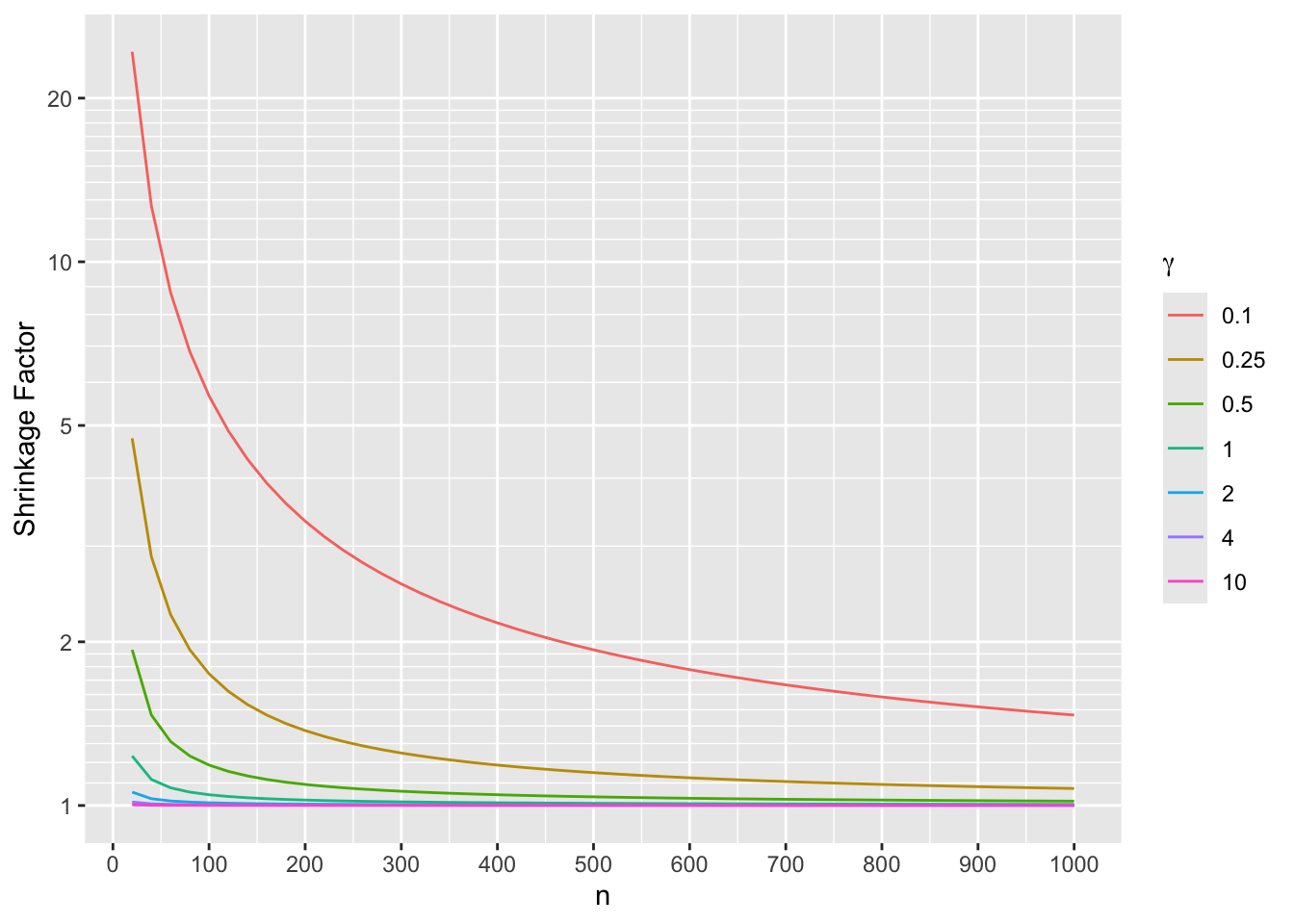One can see for example that when the prior SD for $$\tau$$ is $$\gamma=1$$ the prior causes an estimate of $$\tau$$ to shrink only by only about a factor of 1.25 even for very small sample sizes. By the time there are 200 patients per treatment arm the shrinkage towards PO is not noticeable. The following simulations for 100 patients per arm provide more accurate estimates because formal PMLE is used and the data likelihood is not assumed to be Gaussian. In addition to quantifying the effect of shrinkage caused by different $$\gamma$$ (prior SD of $$\tau$$), we compute the root mean squared errors for estimating log(OR) for $$Y\geq 1$$ and for $$Y=2$$. Code m <- 100 x <- c(rep(0, m), rep(1, m)) nsim <- 500 sds <- c(.0001, 0.1, 0.5, 0.75, 1, 1.5, 2, 4, 10, 50) lsd <- length(sds) gam <- if(ishtml) 'γ' else '$\\gamma\$'
R <- array(NA, c(nsim, lsd, 2),
dimnames=list(NULL, paste0(gam, '=', sds),
c('Y>=1', 'Y=2')))
set.seed(3)
for(i in 1 : nsim) {
y0 <- sample(0:2, m, replace=TRUE, prob=p0)
y1 <- sample(0:2, m, replace=TRUE, prob=p1)
y  <- c(y0, y1)
for(j in 1 : lsd) {
f <- blrm(y ~ x, ~ x, priorsd=1000, priorsdppo=sds[j], method='opt')
k <- coef(f)
# save the two treatment log ORs (for Y>=1 and for Y=2)
R[i, j, 1:2] <- c(k['x'], k['x'] + k['x:y>=2'])
}
}
# For each prior SD compute the two mean log ORs and compare
# truth
cat('True values:\n')
True values:
Code
lors
log OR for Y>=1  log OR for Y=2
0.4418328       0.8109302 
Code
z <- apply(R, 2:3, mean)
z <- cbind(z, Difference=z[, 2] - z[, 1])
z <- cbind(z, 'Shrinkage Factor'=diff(lors) / z[, 'Difference'])
kab(z, caption='Simulated mean log ORs', digits=c(3,3,3,2))
Simulated mean log ORs
Y>=1 Y=2 Difference Shrinkage Factor
γ=1e-04 0.667 0.667 0.000 238693114.13
γ=0.1 0.671 0.672 0.002 233.59
γ=0.5 0.649 0.685 0.036 10.15
γ=0.75 0.626 0.700 0.074 5.00
γ=1 0.601 0.716 0.115 3.21
γ=1.5 0.557 0.746 0.189 1.95
γ=2 0.525 0.768 0.243 1.52
γ=4 0.473 0.807 0.334 1.10
γ=10 0.450 0.825 0.374 0.99
γ=50 0.446 0.829 0.383 0.96
Code
z <- apply(R, 2:3, sd)
kab(z, caption='Simulated SDs of log ORs', digits=3)
Simulated SDs of log ORs
Y>=1 Y=2
γ=1e-04 0.288 0.288
γ=0.1 0.281 0.281
γ=0.5 0.282 0.280
γ=0.75 0.283 0.280
γ=1 0.285 0.279
γ=1.5 0.290 0.281
γ=2 0.294 0.283
γ=4 0.304 0.290
γ=10 0.309 0.294
γ=50 0.310 0.295
Code
rmse <- function(which, actual) {
x <- R[, , which]
apply(x, 2, function(x) sqrt(mean((x - actual)^2)))
}

z <- cbind('Y>=1'=rmse('Y>=1', lors), 'Y=2'=rmse('Y=2', lors))
kab(z, caption='Simulated root MSEs', digits=3)
Simulated root MSEs
Y>=1 Y=2
γ=1e-04 0.365 0.322
γ=0.1 0.362 0.313
γ=0.5 0.350 0.307
γ=0.75 0.338 0.301
γ=1 0.326 0.295
γ=1.5 0.311 0.288
γ=2 0.305 0.286
γ=4 0.305 0.290
γ=10 0.309 0.294
γ=50 0.310 0.296

In a mixed Bayesian/frequentist sense (computing MSE of a posterior mean), the optimum MSE in estimating the two treatment effects (log ORs) was obtained at $$\gamma=2$$. The observed shrinkage factors do not track very well with the approximate ones derived earlier. A better approximation is needed.

See a similar case study in RMS Section 13.3.5. In that example, the sample size is larger and PO is clearly violated.

# Computing Environment

 R version 4.2.1 (2022-06-23)
Platform: x86_64-pc-linux-gnu (64-bit)
Running under: Pop!_OS 22.04 LTS

Matrix products: default
BLAS:   /usr/lib/x86_64-linux-gnu/blas/libblas.so.3.10.0
LAPACK: /usr/lib/x86_64-linux-gnu/lapack/liblapack.so.3.10.0

attached base packages:
 splines   stats4    stats     graphics  grDevices utils     datasets
 methods   base

other attached packages:
 rmsb_0.1.0      VGAM_1.1-6      rms_6.3-1       SparseM_1.81
 Hmisc_4.7-2     ggplot2_3.3.5   Formula_1.2-4   survival_3.4-0
 lattice_0.20-45

To cite R in publications use:

R Core Team (2022). R: A Language and Environment for Statistical Computing. R Foundation for Statistical Computing, Vienna, Austria. https://www.R-project.org/.

To cite the Hmisc package in publications use:

Harrell Jr F (2022). Hmisc: Harrell Miscellaneous. R package version 4.7-2, https://hbiostat.org/R/Hmisc/.

To cite the rms package in publications use:

Harrell Jr FE (2022). rms: Regression Modeling Strategies. https://hbiostat.org/R/rms/, https://github.com/harrelfe/rms.

To cite the rmsb package in publications use:

Harrell F (2022). rmsb: Bayesian Regression Modeling Strategies. R package version 0.1.0, https://hbiostat.org/R/rmsb/.

To cite the VGAM package in publications use:

Yee TW (2015). Vector Generalized Linear and Additive Models: With an Implementation in R. Springer, New York, USA.

To cite the ggplot2 package in publications use:

Wickham H (2016). ggplot2: Elegant Graphics for Data Analysis. Springer-Verlag New York. ISBN 978-3-319-24277-4, https://ggplot2.tidyverse.org.

To cite the survival package in publications use:

Therneau T (2022). A Package for Survival Analysis in R. R package version 3.4-0, https://CRAN.R-project.org/package=survival.